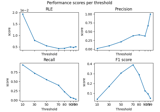# mne.simulation.metrics.cosine_score#

mne.simulation.metrics.cosine_score(stc_true, stc_est, per_sample=True)[source]#

Compute cosine similarity between 2 source estimates.

Parameters
stc_trueinstance of (Vol|Mixed)SourceEstimate

The source estimates containing correct values.

stc_estinstance of (Vol|Mixed)SourceEstimate

The source estimates containing estimated values e.g. obtained with a source imaging method.

per_sample`bool`

If True the metric is computed for each sample separately. If False, the metric is spatio-temporal.

Returns
metric`float` | `array`, shape (n_times,)

The metric. float if per_sample is False, else array with the values computed for each time point.

Notes

New in v1.2.

## Examples using `mne.simulation.metrics.cosine_score`#Compare simulated and estimated source activity

Compare simulated and estimated source activity